# metaBMA: Bayesian Model Averaging for Random- and Fixed-Effects Meta-Analysis

## Overview

Fixed-effects meta-analyses assume that the effect size $$d$$ is identical in all studies. In contrast, random-effects meta-analyses assume that effects vary according to a normal distribution with mean $$d$$ and standard deviation $$\tau$$. Both models can be compared in a Bayesian framework by assuming specific prior distribution for $$d$$ and $$\tau$$. Given the posterior model probabilities, the evidence for or against an effect (i.e., whether $$d = 0$$) and the evidence for or against random effects can be evaluated (i.e., whether $$\tau = 0$$). By using Bayesian model averaging (i.e., inclusion Bayes factors), both types of tests can be performed by marginalizing over the other question. Most importantly, this allows to test whether an effect exists while accounting for uncertainty whether study heterogeneity exists or not.

## Defining and Plotting Priors

To fit a meta-analysis model, prior distributions on the average effect $$d$$ and the heterogeneity $$\tau$$ are required. The package metaBMA leaves the user the freedom to choose from several predefined distributions or even define an owen prior density function. The function prior facilitates the construction and visual inspection of prior distributions to check whether they meet the prior knowledge about the field of interest.

# load package
library("metaBMA")
## Loading required package: Rcpp
## This is metaBMA version 0.6.9
## - Default priors were changed in version 0.6.6.
## - Since default priors may change again, it is safest to specify priors (even when using the defaults).
# load data set
data(towels)

# Half-normal (truncated to > 0)
p1 <- prior("norm", c(mean=0, sd=.3), lower = 0)
p1
## Prior density function (class='prior'): 'norm' (mean=0, sd=0.3) truncated to the interval [0,Inf].
p1(1:3)
##  1.028186e-02 5.940600e-10 5.129732e-22
plot(p1)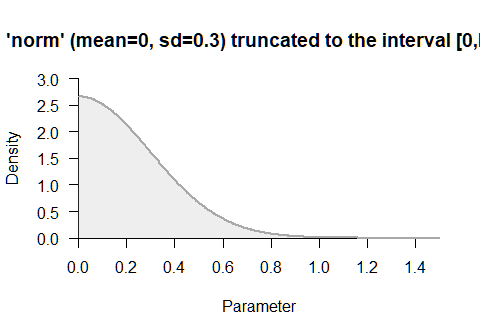# custom prior
p1 <- prior("custom", function(x) x^3-2*x+3, lower = 0, upper = 1)
plot(p1, -.5, 1.5)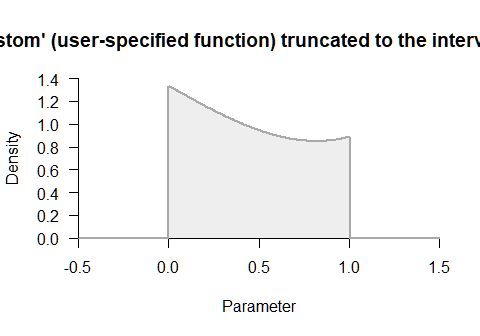## Bayesian Meta-Analysis

The functions meta_fixed() and meta_random() fit Bayesian meta-analysis models. The model-specific posteriors for $$d$$ can then be averaged by bma() and inclusion Bayes factors be computed by inclusion().

The fixed-effects meta-analysis assumes that the effect size is identical across studies. This model requires only one prior distribution for the overall effect $$d$$:

# Fixed-effects
progres <- capture.output(  # suppress Stan progress for vignette
mf <- meta_fixed(logOR, SE, study, towels,
d = prior("norm", c(mean=0, sd=.3), lower=0))
)
mf
## ### Bayesian Fixed-Effects Meta-Analysis ###
##    Prior on d:    'norm' (mean=0, sd=0.3) truncated to the interval [0,Inf].
##
## # Bayes factors:
##            (denominator)
## (numerator) fixed_H0 fixed_H1
##    fixed_H0      1.0   0.0419
##    fixed_H1     23.9   1.0000
##
## # Posterior summary statistics of fixed-effects model:
##    mean    sd  2.5%   50% 97.5% hpd95_lower hpd95_upper n_eff Rhat
## d 0.212 0.075 0.066 0.212 0.361       0.062       0.358    NA   NA
# plot posterior distribution
plot_posterior(mf)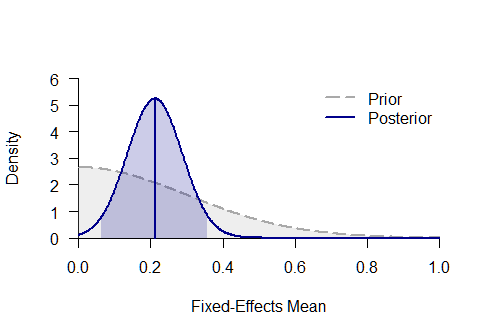In contrast, the random-effects meta-analysis assumes that the effect size varies across studies. Specifically, it is assumed that study effect sizes follow a normal distribution with mean $$d$$ and standard deviation $$\tau$$. This model requires two prior distributions for both parameters:

# Random-effects
progres <- capture.output(  # suppress Stan progress for vignette
mr <- meta_random(logOR, SE, study, towels,
d = prior("norm", c(mean=0, sd=.3), lower=0),
tau = prior("t", c(location=0, scale=.3, nu=1), lower=0),
iter = 1500, logml_iter = 2000, rel.tol = .1)
)
mr  
## ### Bayesian Random-Effects Meta-Analysis ###
##    Prior on d:      'norm' (mean=0, sd=0.3) truncated to the interval [0,Inf].
##    Prior on tau:    't' (location=0, scale=0.3, nu=1) truncated to the interval [0,Inf].
##
## # Bayes factors:
##            (denominator)
## (numerator) random_H0 random_H1
##   random_H0      1.00     0.264
##   random_H1      3.78     1.000
##
## # Posterior summary statistics of random-effects model:
##      mean    sd  2.5%   50% 97.5% hpd95_lower hpd95_upper  n_eff  Rhat
## d   0.197 0.084 0.042 0.196 0.364       0.035       0.355 1789.2 1.001
## tau 0.116 0.104 0.003 0.090 0.392       0.000       0.315 1727.3 1.001
# plot posterior distribution
plot_posterior(mr, main = "Average effect size d")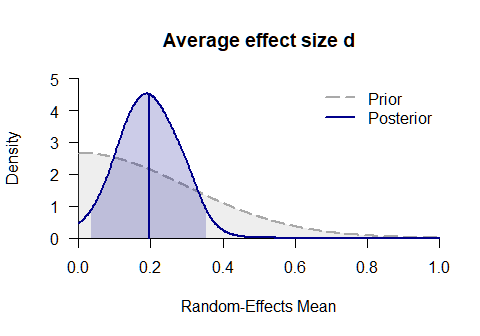plot_posterior(mr, "tau", main = "Heterogeneity tau")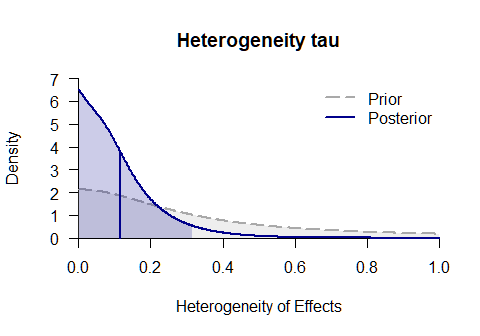## Model-Averaging for Meta-Analysis

The most general functions in metaBMA are meta_bma() and meta_default(), which fit random- and fixed-effects models, compute the inclusion Bayes factor for the presence of an effect and the averaged posterior distribution of the mean effect $$d$$ (which accounts for uncertainty regarding study heterogeneity).

mb <- meta_bma(logOR, SE, study, towels,
d = prior("norm", c(mean=0, sd=.3), lower=0),
tau = prior("t", c(location=0, scale=.3, nu=1), lower=0),
iter = 1500, logml_iter = 2000, rel.tol = .1)
mb
## ### Meta-Analysis with Bayesian Model Averaging ###
##    Fixed H0:  d = 0
##    Fixed H1:  d ~ 'norm' (mean=0, sd=0.3) truncated to the interval [0,Inf].
##    Random H0: d   = 0,
##               tau ~  't' (location=0, scale=0.3, nu=1) truncated to the interval [0,Inf].
##    Random H1: d   ~ 'norm' (mean=0, sd=0.3) truncated to the interval [0,Inf].
##               tau ~ 't' (location=0, scale=0.3, nu=1) truncated to the interval [0,Inf].
##
## # Bayes factors:
##            (denominator)
## (numerator) fixed_H0 fixed_H1 random_H0 random_H1
##   fixed_H0      1.00   0.0419     0.433     0.114
##   fixed_H1     23.87   1.0000    10.330     2.730
##   random_H0     2.31   0.0968     1.000     0.264
##   random_H1     8.74   0.3663     3.784     1.000
##
## # Bayesian Model Averaging
##   Comparison: (fixed_H1 & random_H1) vs. (fixed_H0 & random_H0)
##   Inclusion Bayes factor: 9.851
##   Inclusion posterior probability: 0.908
##
## # Model posterior probabilities:
##           prior posterior logml
## fixed_H0   0.25    0.0278 -5.58
## fixed_H1   0.25    0.6644 -2.40
## random_H0  0.25    0.0643 -4.74
## random_H1  0.25    0.2434 -3.41
##
## # Posterior summary statistics of average effect size:
##           mean    sd  2.5%   50% 97.5% hpd95_lower hpd95_upper  n_eff  Rhat
## averaged 0.208 0.079 0.046 0.208 0.366       0.042       0.361     NA    NA
## fixed    0.218 0.072 0.077 0.216 0.360       0.085       0.367  770.1 1.005
## random   0.195 0.092 0.018 0.194 0.382       0.005       0.352 1685.0 1.004
plot_posterior(mb, "d", -.1, 1.4)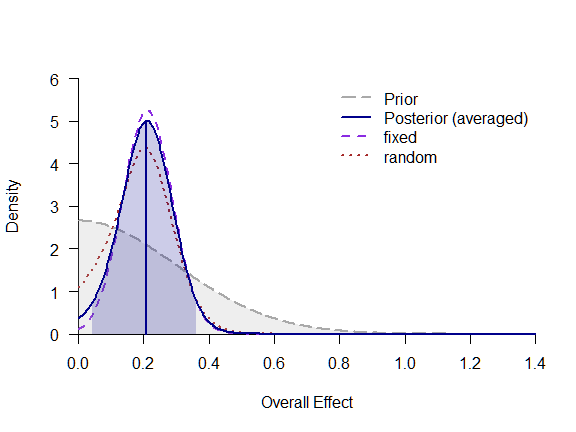plot_forest(mb)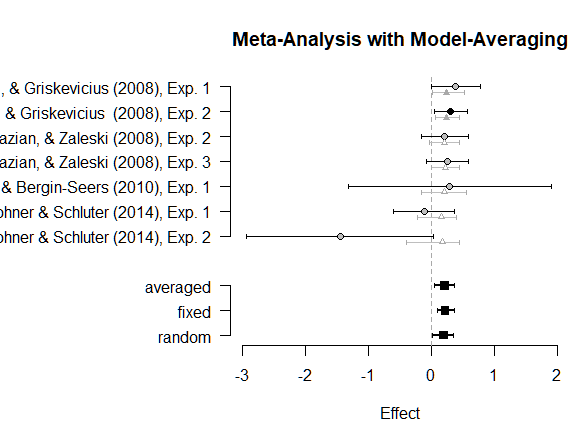## Predicted Bayes Factor for New Study

Often, it is of interest to judge how much additional evidence future studies can contribute to the present knowledge. Conditional on the outcome of the model averaging for meta-analysis, the function predicted_bf() samples new data sets from the posterior and performs model selection for each replication. Thereby, a distribution of predicted Bayes factors is obtain that represents the expected evidence one expects when running a new study. The following example is not executed since it requires time-intensive computations:

mp <- predicted_bf(mb, SE = .2, sample = 30)
plot(mp)